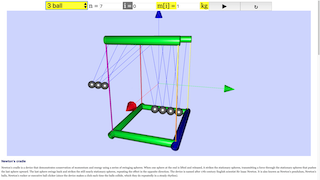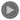Intro Page

Newton's cradle is a device that demonstrates conservation of momentum and energy using a series of swinging spheres. When one sphere at the end is lifted and released, it strikes the stationary spheres, transmitting a force through the stationary spheres that pushes the last sphere upward. The last sphere swings back and strikes the still nearly stationary spheres, repeating the effect in the opposite direction. The device is named after 17th-century English scientist Sir Isaac Newton. It is also known as Newton's pendulum, Newton's balls, Newton's rocker or executive ball clicker (since the device makes a click each time the balls collide, which they do repeatedly in a steady rhythm).

A typical Newton's cradle consists of a series of identically sized metal balls suspended in a metal frame so that they are just touching each other at rest. Each ball is attached to the frame by two wires of equal length angled away from each other. This restricts the pendulums' movements to the same plane.

## Controls

The first comboBox allows eay selection of number of balls in the Newton's cradle

1 ball to the left

2 balls to the left

3 balls to the left

4 balls to the left

5 balls to the left

6 balls to the left

7 balls to the left

1 ball 1 raises 1 ball to the left and 1 ball to the right

2 ball 1 raises 2 ball to the left and 1 ball to the right

2 ball 2 raises 2 balls to the left and 2 balls to the right

3 ball 3 raises 3 balls to the left and 3 balls to the right

n is the number of balls allowed

i is the index of the ball to change

for which m[i] is the mass of the index ball

play button starts the simulation

reset button starts the simulation at afresh

## Physics explanation

Newton's cradle can be modeled fairly accurately with simple mathematical equations with the assumption that the balls always collide in pairs.

The motion of the balls are modelled using dθ[i]dt=ω[i]$\frac{d\theta \left[i\right]}{dt}=\omega \left[i\right]$

where θ$\theta$ is the angle of the ball

ω$\omega$ is the angular velocity

i represents each element of the ball

t is time

the second order differential equation is d(ω[i])dt=gsin(θ[i])LkLω[i]$\frac{d\left(\omega \left[i\right]\right)}{dt}=-\frac{g\ast sin\left(\theta \left[i\right]\right)}{L}-k\ast L\ast \omega \left[i\right]$

where g is gravitational constant 9.81 m/s2$m/{s}^{2}$

L is the length of the inextensible string connecting the ball to the support for rotational motion

k is a resistant coefficient to rotational motion ω$\omega$

## Events of the ordinary differential equations

In a state event, a TOLERANCE =1.0e-6 is defined, a loop to check through each ball i, where a check of the θ[i]$\theta \left[i\right]$ is greater than θ[i+1]$\theta \left[i+1\right]$ plus the TOLERANCE, if true, return the balls to their closed up angular position θ[i+1]θ[i]$\theta \left[i+1\right]-\theta \left[i\right]$. If false, do nothing.

## Fixed Relationship Calculations

A for loop over i=0 to i less than number of balls to calculate the following

dx[i]=L(sinθ[i])$dx\left[i\right]=L\ast \left(sin\theta \left[i\right]\right)$ where dx[i] is the change is x[i] position

dy[i]=Lcos(θ[i])$dy\left[i\right]=-L\ast cos\left(\theta \left[i\right]\right)$ where dy[i] is the change in y[i] position

x[i]=xc[i]+dx[i] where xc[i] is each balls centre or equilibrium x position

y[i]=ymax+dy[i] where ymax is the support y position

vx[i]=Lω[i]cos(θ[i])$vx\left[i\right]=L\ast \omega \left[i\right]\ast cos\left(\theta \left[i\right]\right)$ where vx[i] is the x direction linear velocities of each ball

vy[i]=Lω[i]sin(θ[i])$vy\left[i\right]=L\ast \omega \left[i\right]\ast sin\left(\theta \left[i\right]\right)$ where vy[i] is the y direction linear velocities of each ball

### Translations

Code Language Translator Run### Software Requirements

SoftwareRequirements

 Android iOS Windows MacOS with best with Chrome Chrome Chrome Chrome support full-screen? Yes. Chrome/Opera No. Firefox/ Samsung Internet Not yet Yes Yes cannot work on some mobile browser that don't understand JavaScript such as..... cannot work on Internet Explorer 9 and below

### CreditsFu-Kwun Hwang and lookang (This email address is being protected from spambots. You need JavaScript enabled to view it.); lookang; Francisco Esquembre

### Description

Newton's cradle, named after Sir Isaac Newton, is a device that demonstrates conservation of momentum and energy via a series of swinging spheres.

Construction in 3D
A typical Newton's cradle consists of a series of identically sized metal balls suspended in a metal frame so that they are just NOT touching each other at rest. Each ball is attached to the frame by two wires of equal length angled away from each other. This restricts the pendulums' movements to the same plane.

# Mathematical Model:

the simplified equations of motion is ODE for a single mass(pendulum) are:
d (cta)/dt = omega
d(omega)/dt = -g*sin(cta)/L

when extended to n array of masses, for for(int i=0;i<n;i++){
d (cta[i])/dt = omega[i]
d(omega[i])/dt = -g*sin(cta[i])/L  // simplified add here from air drag
}
the zero condition type as "State event" with the following code

// start of code for zero condition
double min = TOLERANCE;
for(int i=0;i<n-1;i++){
if(cta[i]>cta[i+1]+TOLERANCE){//<TOLERANCE){// collision between i and i+1;
cid=i;
return cta[i+1]-cta[i];
}
}
//end of code for zero condition

The action is to assumes the collision are modeled by perfectly elastic collision in one dimension with angular momentum conserved
the code is as below.
// start of code for action
m1=m[cid];
m2=m[cid+1];
v1=L*omega[cid];
v2=L*omega[cid+1];
va=((m1-m2)*v1+2*m2*v2)/(m1+m2);// velocity after collision
vb=(2*m1*v1+(m2-m1)*v2)/(m1+m2);
omega[cid]=va/L;// back to omega
omega[cid+1]=vb/L;
//end of code for action

# Design features:

currently, the simulation allows of exploration of n max = 7 masses with each mass m[i] that can be vary from the slider control from 1 to 5 kg.
gravitational constant downward g = 9.81 m/s^2
Length of pendulums, L
k coefficicent of air resistance model by equation F = k*omega

The usabilty control:
To move the balls, move the cursor over to the mass, click and hold the left mouse and drag the mouse to lift the masses. note that all masses are selectable and movable to a new height along the each path of the pendulums swing.

# Design Views:

there are 2 types views available selectable by the check-boxes 2D and 3D,
2D world view
3D world view
hint:
may be useful to observe the transfer of linear momentum from ball to ball by pressing the step button to aid understanding of momentum transfer during collisions
may be useful to observe the scientific representations of kinetic energies KE[i], gravitational potential energies PE[i] and total mechanical energies TE[i]
graph of scientific representations of kinetic energies KE total, gravitational potential energies PE total and total mechanical energies TE total

# Exercises:

select n =2 for a simple 2 ball system for the experiment.

Pull and release one ball. Note the results and explain mathematically in terms of conservation of engergy and momentum.
m1 u1 + m1 u1 = m1 v1+ m2 v2
1/2 m1 u1^2 + 1/2 m2 u2^2 = 1/2 m1 v1^2 + 1/2 m2 v2^2
record your observations and discuss if the two equations above can account for the observations

select n = 3 , 4, 5, 6, 7 and repeat the experiment above.
Do the results meet your expectations?
hint: can you conclude that the just before and just after collisions
momentum in = momentum out
kinetic energies in = kinetic energies out.
the clue lies in the conservation of momentum and kinetic energies just before and after collisions.

Discuss what are the differences between this computer model and real life apparatus.
hint: the newton's cradle motion will continue in this back and fro motion until all energies are lost to damping due to air resistance, friction, sound and vibrations.

In this simulation the default is all balls have the same mass. What would occur if this was not the case?

Please submit your remix model that model features that are not available in the existing virtual lab and share your model with the world through NTNUJAVA Virtual Physics Laboratory http://www.phy.ntnu.edu.tw/ntnujava/index.php?board=28.0. Impacting the world with your model now.

### Credits:

The Newton's Cradle Model was created by Fu-Kwun Hwang, customized by Loo Kang WEE, using the Easy Java Simulations (EJS) version 4.3.3.2 authoring and modeling tool.  An applet version of this model is available on the NTNU website < http://www.phy.ntnu.edu.tw/ntnujava/index.php?topic=2195.0 >.

You can examine and modify this compiled EJS model if you run the model (double click on the model's jar file), right-click within a plot, and select "Open EJS Model" from the pop-up menu.  You must, of course, have EJS installed on your computer.  Information about EJS is available at: <http://www.um.es/fem/Ejs/> and in the OSP comPADRE collection <http://www.compadre.org/OSP/>.

# Testimonials (0)

There are no testimonials available for viewing. Login to deploy the article and be the first to submit your review!